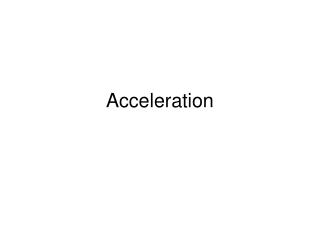DownloadDownload PresentationAcceleration

# Acceleration

Télécharger la présentation## Acceleration

- - - - - - - - - - - - - - - - - - - - - - - - - - - E N D - - - - - - - - - - - - - - - - - - - - - - - - - - -
##### Presentation Transcript

1. Acceleration

2. Acceleration = change in velocity

3. Changing velocity • When you change velocity- either speed or direction- you have acceleration • Remember our motion diagrams? • When magnitude of velocity changes, distance between dots changes

4. Speed and Acceleration • When could you have acceleration but have constant speed?

5. Acceleration is a Vector! • This means it has both magnitude and direction • Rule of thumb: if acceleration is in same direction as velocity= speeding up • If acceleration is in opposite direction of velocity= slowing down

6. Average Acceleration • a=v/ t • Units are m/s2 • Because acceleration is a vector, the sign means direction • Remember the rule of thumb: same sign as velocity then speeding up

7. Velocity-Time Graphs • If you graph velocity vs. time, the slope (or rise/run) would be the v/ t • So slope of a v-t graph=acceleration

8. Meaning of v-t graphs • Flat line=constant velocity= no acceleration • the position of the line above or below the x axis has meaning • In this case, is velocity + or - and how can you tell?

9. Position of line above/below x axis denotes + or - velocity

10. Slope= acceleration but beware of sign of velocity!

11. Using graphs to find acceleration • Rise/run= v/ t

12. Problem solving • Acceleration =v/ t • Remember your problem-solving steps- it becomes very important to sketch the problem- if you sketch the velocity and acceleration vectors, it will help you determine signs • Check your units- they should easily cancel to give the correct units for your answer

13. Example: • A race car’s velocity increases from 4.0 m/s to 36 m/s over a 4.0s time period. What is the average acceleration? • Acceleration =v/ t • a=(36.0m/s-4.0m/s) = 8.0 m/s2 4.0s

14. Practice Problems • P. 64 #7, 8

15. Honors: solving for velocity • You can rearrange the average acceleration equation to solve for initial or final velocity: • a= v/ t • v=a t • vf-vi=a t • vf=vi+a t

16. Honors: practice • p. 65 #18

17. Velocity-Time Graphs and Displacement • If the slope of a v-t graph shows acceleration, how can we find the displacement? • The area under a v-t graph is the displacement for that segment

18. Calculating area • Remember area calculations? • Area of a rectangle= • d=length * width • d= t* v • Area of a triangle= •  d=1/2 base*height • d=1/2 t * v

19. Honors: kinematics equations • These area formulae can be rearranged to find final position of an object with constant acceleration • Only works with constant acceleration

20. Kinematics Equations Assumptions • Object starts at x0=0 so x=(x1-x0)=x • Acceleration is constant • Initial velocity = v0 • Since a is constant, v=1/2(v1+v0)

21. Kinematics Equations • v=v0+at • x= v0t + 1/2 at2 • V2=v02+2a(x-x0) • Plus we know (or can figure out from units) • x=v t • v=a t

22. How do I know which one to use? • Each equation is missing one variable- if you write down what you know at the start of a problem (including what is asked for) you will end up with something missing- neither given nor asked for. • The equation that will work is missing this variable.

23. So what’s missing? • v=v0+at x • x= v0t + 1/2 at2 v • v2=v02+2a(x-x0) t • s=v t a • v=a t s

24. Reminder • DO NOT IGNORE the problem-solving steps!!! They are there to help you. Write down what you know and what you are solving for first! • Always check units as you set it up and when you solve.

25. Examples • An object with an initial velocity of 4 m/s moves along a straight line under constant acceleration. Three seconds later, its velocity is 14 m/s. How far did it travel during this time? • v0= 4 m/s • t= 3 s • v1 = 14 m/s • x=? • What’s missing? a

26. Example- continued • Since a is missing we use: x=v t • Constant acceleration so v=1/2(v+v0) • s=1/2(4m/s + 14m/s)(3s)=27m • Are your units correct? Does the answer make sense? • Leaving off units in your final answer WILL COST YOU POINTS!